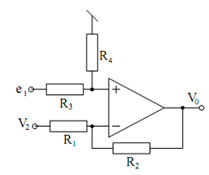## Finite Gain Differential Amplifiers Assignment Help

Assignment Help: >> Basic Analog Circuits using Ideal Op-amps - Finite Gain Differential Amplifiers

Finite Gain Differential Amplifiers:

In the 3-port VCVS of Figure, if a potential divider is added at the input, the circuit of Figure is achieved for which we get :

V1  =     (R4/ R3  + R4)    e1

Combining Eqs. (58) and (53), we get

V0  = ( 1+ (R2/R1) /(   1+ (R3/R4)   e -( R2 /R1) VFigure: Finite Gain Differential Amplifier

Letting R2 /R1 = (1+ (R2/R1) /(1+ (R3/R4)   - K

The circuit realizes a K-gain differential amplifier for which the needed resistor values are found to be

R2 = k R1, R4 = KR3